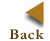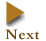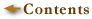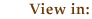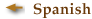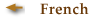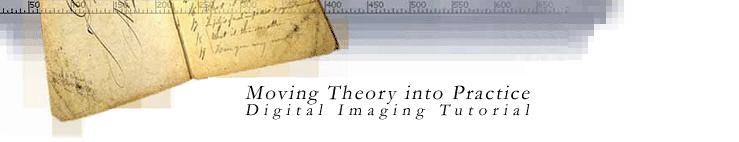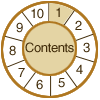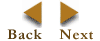1. Basic  Terminology

Key Concepts

digital images
resolution
pixel dimensions
bit depth
dynamic range
file size
compression
file formats

BIT DEPTH is determined by the number of bits used to define each pixel. The greater the bit depth, the greater the number of tones (grayscale or color) that can be represented. Digital images may be produced in black and white (bitonal), grayscale, or color.

A bitonal image is represented by pixels consisting of 1 bit each, which can represent two tones (typically black and white), using the values 0 for black and 1 for white or vice versa.

A grayscale image is composed of pixels represented by multiple bits of information, typically ranging from 2 to 8 bits or more.

 Example: In a 2-bit image, there are four possible combinations: 00, 01, 10, and 11. If "00" represents black, and "11" represents white, then "01" equals dark gray and "10" equals light gray. The bit depth is two, but the number of tones that can be represented is 2 2 or 4. At 8 bits, 256 (2 8 ) different tones can be assigned to each pixel.

A color image is typically represented by a bit depth ranging from 8 to 24 or higher. With a 24-bit image, the bits are often divided into three groupings: 8 for red, 8 for green, and 8 for blue. Combinations of those bits are used to represent other colors. A 24-bit image offers 16.7 million (2 24 ) color values. Increasingly scanners are capturing 10 bits or more per color channel and often outputting 8 bits to compensate for "noise" in the scanner and to present an image that more closely mimics human perception.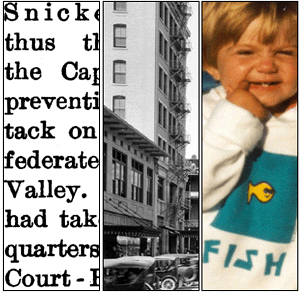Bit Depth: Left to right - 1-bit bitonal, 8-bit grayscale, and 24-bit color images.

Binary calculations for the number of tones represented by common bit depths:

1 bit (21) = 2 tones
2 bits (22) = 4 tones
3 bits (23) = 8 tones
4 bits (24) = 16 tones
8 bits (28) = 256 tones
16 bits (216) = 65,536 tones
24 bits (224) = 16.7 million tones

© 2000-2003 Cornell University Library/Research Department